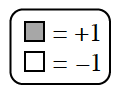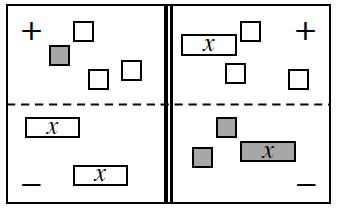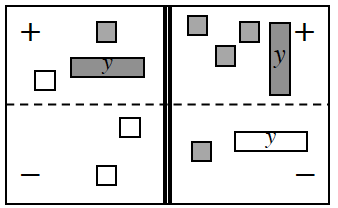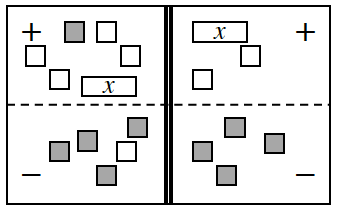### Home > INT1 > Chapter A > Lesson A.1.9 > ProblemA-96

A-96.1.The expression for the left side is $1 − 3 − \left(2x\right)$. The expression for the right side is $−x − 3 −\left(2 + x\right)$. Simplify each side by flipping tiles from the negative to the positive region and removing zero pairs. Record your work.

$2x − 2 = −2x − 5$

$4x = −3$

$x\ =\ -\frac{3}{4}$

1.The simplified expression for the left side is$y + 2$, for the right side is $2y + 2$.

1.